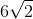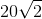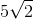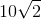# High School Math : Squares

## Example Questions

### Example Question #11 : Squares

A half circle has an area of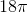. What is the area of a square with sides that measure the same length as the diameter of the half circle?

81

72

144

108

36

144

Explanation:

If the area of the half circle is, then the area of a full circle is twice that, or.

Use the formula for the area of a circle to solve for the radius:

36π = πr2

r = 6

If the radius is 6, then the diameter is 12. We know that the sides of the square are the same length as the diameter, so each side has length 12.

Therefore the area of the square is 12 x 12 = 144.

### Example Question #40 : Squares

Eric has 160 feet of fence for a parking lot he manages. If he is using all of the fencing, what is the area of the lot assuming it is square?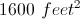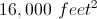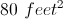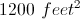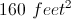Explanation:

The area of a square is equal to its length times its width, so we need to figure out how long each side of the parking lot is. Since a square has four sides we calculate each side by dividing its perimeter by four.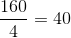Each side of the square lot will use 40 feet of fence.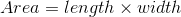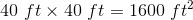.

### Example Question #5 : Quadrilaterals

A circle with a radius 2 in is inscribed in a square. What is the perimeter of the square?

32 in

16 in

24 in

12 in

28 in

16 in

Explanation:

To inscribe means to draw inside a figure so as to touch in as many places as possible without overlapping. The circle is inside the square such that the diameter of the circle is the same as the side of the square, so the side is actually 4 in.  The perimeter of the square = 4s = 4 * 4 = 16 in.

### Example Question #2 : How To Find The Perimeter Of A Square

Square X has 3 times the area of Square Y.  If the perimeter of Square Y is 24 ft, what is the area of Square X, in sq ft?

54

108

112

72

144

108

Explanation:

Find the area of Square Y, then calculate the area of Square X.

If the perimeter of Square Y is 24, then each side is 24/4, or 6.

A = 6 * 6 = 36 sq ft, for Square Y

If Square X has 3 times the area, then 3 * 36 = 108 sq ft.

### Example Question #3 : How To Find The Perimeter Of A Square

A square has an area of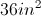.  If the side of the square is reduced by a factor of two, what is the perimeter of the new square?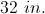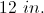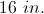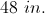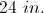Explanation:

The area of the given square is given by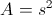so the side must be 6 in.  The side is reduced by a factor of two, so the new side is 3 in.  The perimeter of the new square is given by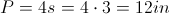.

### Example Question #4 : How To Find The Perimeter Of A Square

A square garden has an area of 64 square feet. If you add 3 feet to each side, what is the new perimeter of the garden?

121

25

32

20

44

44

Explanation:

By finding the square root of the area of the garden, you find the length of one side, which is 8. We add 3 feet to this, giving us 11, then multiply this by 4 to get 44 feet for the perimeter.

### Example Question #5 : How To Find The Perimeter Of A Square

The area of the shaded region of a square is 18. What is the perimeter of the square?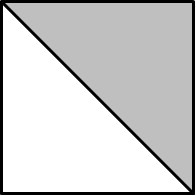28

24

36

20

24

Explanation:

The area of the shaded region, which covers half of the square is 18 meaning that the total area of the square is 18 x 2, or 36. The area of a square is equal to the length of one side squared. Since the square root of 36 is 6, the length of 1 side is 6. The perimeter is the length of 1 side times 4 or 6 x 4.

### Example Question #6 : How To Find The Perimeter Of A Square

The area of a square is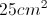.  If the square is enlarged by a factor of 2, what is the perimeter of the new square?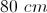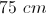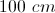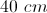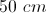Explanation:

The area of a square is given byso we know the side is 5 cm.  Enlarging by a factor of two makes the new side 10 cm.  The perimeter is given by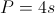, so the perimeter of the new square is 40 cm.

### Example Question #1 : How To Find The Perimeter Of A Square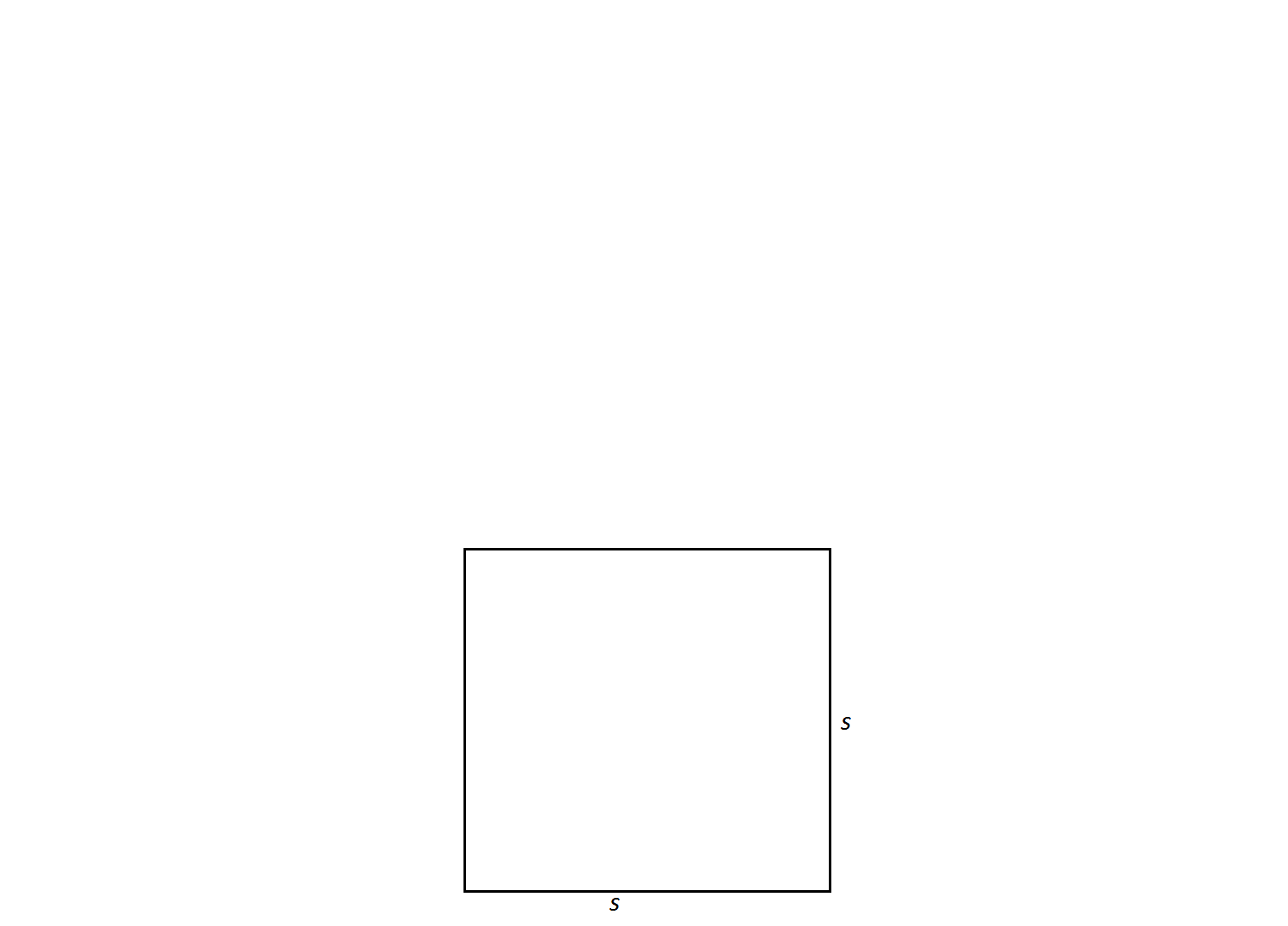A square has a side length of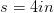. Find its perimeter.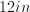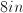Not enough information to solve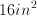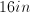Explanation:

In order to find the perimeter of a square, one must add up all of its side lengths. The side lengths of a square are all equal; therefore, the formula for the perimeter of a square is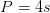.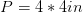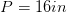Remember perimeter should be expressed in single units not units squared. Thus,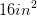is an incorrect answer.

### Example Question #7 : How To Find The Perimeter Of A Square

The diagonal of a square has a length of 10 inches. What is the perimeter of the square in inches squared?